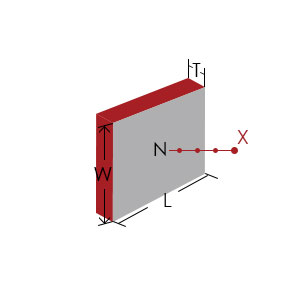# FROM DESIGN TO PRODUCT

We put our customers first• Gauss Calculator
• Disc Shape
• Square Shape
• Weight Calculator
• NdFeB Magnet
• SmCo Magnet
• AlNiCo Magnet
• Ferrite Magnet
• Request a Quote

### NdFeB Disk Gauss Calculator

Fill In This Form
• Magnet Performance：
• Remanence Br：
• Gauss
• Diameter d：
• mm
• Thickness h：
• mm
Note: Please fill in the specific parameters of the magnet you need, you can calculate the corresponding value of the magnet. You can also compare the following magnet diagram.View Results
• Magnetic field intensity Bp：
• Gauss
Remarks：
1.The calculated result is the magnetic force of magnet surface center and the magnetization get through thickness .
2.The magnetic force accosiate with residual industion ,so if the magnet size and residual industion are same, whatever N,M,H,SH,UH,EH or AH, the surface magnetic field will be same.

### NdFeB Square Gauss Calculator

Fill In This Form
• Magnet Performance:
• Remanence Br：
• Gauss
• Length l：
• mm
• Width w：
• mm
• Thickness h：
• mm
Note: Please fill in the specific parameters of the magnet you need, you can calculate the corresponding value of the magnet. You can also compare the following magnet diagram.View Results
• Magnetic field intensity Bp：
• Gauss
Remarks：
1.The calculated result is the magnetic force of magnet surface center and the magnetization get through thickness .
2.The magnetic force accosiate with residual industion ,so if the magnet size and residual industion are same, whatever N,M,H,SH,UH,EH or AH, the surface magnetic field will be same.

### NdFeB Magnet

#### Disc

• Diameter：
• mm
• Thickness：
• mm
• Weight：
• g
• Number:
• Total weight:
• g

#### Ring

• External Diameter:
• mm
• Internal Diameter:
• mm
• Height:
• mm
• Weight：
• g
• Number:
• Total weight:
• g

#### Square

• Length:
• mm
• Width:
• mm
• Height：
• mm
• Weight：
• g
• Number:
• Total weight:
• g

#### Sphere

• Diameter:
• mm
• Weight：
• g
• Number:
• Total weight:
• g

### SmCo Magnet

#### Disc

• Diameter：
• mm
• Thickness：
• mm
• Weight：
• g
• Number:
• Total weight:
• g

#### Ring

• External Diameter:
• mm
• Internal Diameter:
• mm
• Height:
• mm
• Weight：
• g
• Number:
• Total weight:
• g

#### Square

• Length:
• mm
• Width:
• mm
• Height：
• mm
• Weight：
• g
• Number:
• Total weight:
• g

### AlNiCo Magnet

#### Disc

• Diameter：
• mm
• Thickness：
• mm
• Weight：
• g
• Number:
• Total weight:
• g

#### Ring

• External Diameter:
• mm
• Internal Diameter:
• mm
• Height:
• mm
• Weight：
• g
• Number:
• Total weight:
• g

#### Square

• Length:
• mm
• Width:
• mm
• Height：
• mm
• Weight：
• g
• Number:
• Total weight:
• g

### Ferrite Magnet

#### Disc

• Diameter：
• mm
• Thickness：
• mm
• Weight：
• g
• Number:
• Total weight:
• g

#### Ring

• External Diameter:
• mm
• Internal Diameter:
• mm
• Height:
• mm
• Weight：
• g
• Number:
• Total weight:
• g

#### Square

• Length:
• mm
• Width:
• mm
• Height：
• mm
• Weight：
• g
• Number:
• Total weight:
• g